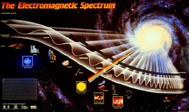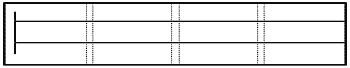Featured Resources
Space Math @ NASA
NSO Tests 2023
- MIT Invitational
Informal Education
Education Collaborations
Web Shortcuts
High School Student Handout

#### Logarithmic and Linear Models of the Electromagnetic SpectrumModels of the electromagnetic spectrum similar to the one pictured above are used extensively in textbooks and on posters, and like all other models it contains distortions. The model is extremely useful for showing the frequencies of the different bands of electromagnetic radiation (EMR), and the relationships between frequency and wavelength. However, this is a logarithmic model, and severely distorts the actual width of the different bands of radiation. The result is that looking at this model gives you the wrong idea that the radio band is very large compared to the X-ray band, for example. You are going to construct both models, logarithmic and linear, on the same chart and compare the two models.

1. Use either the template provided or the following instructions to construct the diagram shown below. Tape four pieces of 8 1/2" by 11" paper together end-to-end so that their long sides are on the bottom. The pieces of paper should overlap by 3 cm. Then draw a line down the left side of the chart about 2 cm from the edge (see diagram below). From the line you have just drawn, draw two horizontal lines extending to the right across the pages: one line 8 cm from the top of the chart, and the other line 10 cm below the first horizontal line.2. The top line will be used to plot the logarithmic model. Along this line, mark off 24 2-cm intervals from the vertical line you drew. Starting at 2 cm, label each interval with increasing powers of ten, from 101 to 1024. These numbers represent the frequency in Hertz of the electromagnetic spectrum. Use the information from the Frequency Range Table on the next page to divide your model into the individual bands of electromagnetic radiation. [Use the entire visible band, not the individual colors.]

EMR Bands Frequency Range
(Hertz)
Radio & Microwave Near 0 to 3.0 x 1012
Infrared 3.0 x 1012 to 4.6 x 1014
Visible 4.6 x 1014 to 7.5 x 1014
Red 4.6 x 1014 to 5.1 x 1014
Orange 5.1 x 1014 to 5.6 x 1014
Yellow 5.6 x 1014 to 6.1 x 1014
Green 6.1 x 1014 to 6.5 x 1014
Blue 6.5 x 1014 to 7.0 x 1014
Violet 7.0 x 1014 to 7.5 x 1014
Ultraviolet 7.5 x 1014 to 6.0 x 1016
X-ray 6.0 x 1016 to 1.0 x 1020
Gamma Ray 1.0 x 1020 to...

3. Before you can construct the linear model, it is necessary to convert the frequencies that you used for the logarithmic model. Those numbers simply told you the range of frequencies, or amount of energy, that each of the bands of EMR covers within the spectrum. Now we want to compare the width of each of the individual bands of radiation relative to each other. We can do this by converting all of the bands of EMR to the same frequency range. We will arbitrarily select the frequency range of the visible band, 1014. Convert the frequency numbers for all bands (except visible) in the Frequency Range ConversionTable below to 1014 and record them in the table. [There is a tutorial on converting exponents on the last page.]

EMR Bands Frequency Range
(Hertz)
Infrared
Visible 4.6 x 1014 to 7.5 x 1014
Red 4.6 x 1014 to 5.1 x 1014
Orange 5.1 x 1014 to 5.6 x 1014
Yellow 5.6 x 1014 to 6.1 x 1014
Green 6.1 x 1014 to 6.5 x 1014
Blue 6.5 x 1014 to 7.0 x 1014
Violet 7.0 x 1014 to 7.5 x 1014
Ultraviolet
X-ray
Gamma Ray

4. Mark off 10 10-cm intervals from the vertical line. Starting at the first interval, label each mark as a whole number times 1014, from 1 x 1014 to 10 x 1014. Label the bottom of your model "Frequency in Hertz." You can now plot some of the 1014 frequencies you calculated on the bottom line of your constructed model. Plot the individual colors of the visible spectrum and color them. Compare the two models. Do the results surprise you?
5. How far does the ultraviolet band extend? Calculate the width of the ultraviolet band. What do you think you would need to measure the distance to the end of the UV part of the EMR?
6. X-rays are the next band of radiation. Calculate the distance from the end of the ultraviolet band to the end of the X-ray band. What do you think you would need to measure the distance to the end of the X-Ray band?
7. Based on your results for the width of the X-ray band, what would be your estimate for the width of the gamma ray band of radiation? What would you need to measure the distance?
8. Compare and contrast the distortions between the two models. What is the advantage in using the logarithmic model? What is the advantage in using the linear model? Can you think of any other ways to model the electromagnetic spectrum?

#### Using Scientific Notation

Methods such as abbreviations and acronyms are used to make long words or long phrases easier to write. For example, in this activity the acronym EMR is sometimes used instead of the words "ElectroMagnetic Radiation". The acronyms NASA is used instead of the words "National Aeronautics and Space Administration". The same technique is used with numbers, large numbers are shortened by using scientific notation (exponents.) For example, the number 1,000,000 written in scientific notation, or exponential form, is 1 x 106

100 = 10 x 10 = 1 x 102
1000 = 10 x 10 x 10 = 1 x 103
100,000 = 10 x 10 x 10 x 10 x 10 = 1 x 105

What if we wanted to express the number 100 [1 x 102] as an exponent of 103? The difference between 102 and 103 is one exponent so we would move the decimal one place. Since we are expressing a number as a larger exponent, the decimal place is moved one place to the left, so 1 x 102 becomes 0.1 x 103.

What if we wanted to express the number 100,000 [1 x 105] as an exponent of 103?

The difference between 105 and 103 is two exponents so we would move the decimal two places. Since we are expressing a number as a smaller exponent, the decimal place is moved two places to the right, so 1 x 105 becomes 100 x 103.

Model Construction Template pdf

Modeling the Electromagnetic Spectrum: Middle School
Modeling the Electromagnetic Spectrum: High School